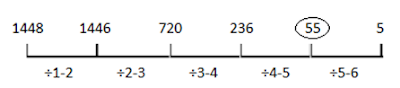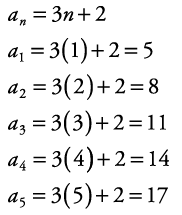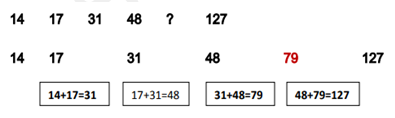## Sequence and Series Definitions, formulas, and shortcuts

By: Admin | Posted on: Apr 9, 2020

Today we will cover sequence and series topic, it is an important topic for almost all competitive exams. When we observe the questions in old competitive exams like SSC, IBPS, SBI PO, CLERK, RRB, and other entrance exams, there are mostly in form of a missing number or complete the pattern series. This topic or section may carry up to 5 marks in competitive exams.

By the end of this article. We can find all questions related to sequence and series in the following section

## Sequence

A succession of numbers a1, a2, a3, a4…an, formed according to certain rule is known as a sequence.

### What are the Sequences?

A sequence is a function whose domain is the set of N natural numbers and ranges to a subset of real numbers or complex numbers. The different terms of a sequence are usually denoted bya1, a2, a3 … or by t1,t2,t3…

The subscript denotes the position of the term in the sequence. The term at the nth place of a sequence i.e., tn is called the general term of the Sequence

In general, we have two types of sequences

• Finite sequences
• Infinite sequences

Finite sequences are those sequences that have a limited number of terms and it may or may not be conditional.

Example 1, 2,3,4,5.

Infinite sequences are those sequences which do not have a limited number of terms it can be extended and not have the finite scope.

Example 1, 2, 3, 4…, n. where n is the nth term.

We will study more about the nth term in the following sections.

Important types of series and sequences

1. Arithmetic Sequence
2. Geometric Sequence
3. Harmonic Sequences
4. Triangular Numbers
5. Square Numbers
6. Cube Numbers
7. Fibonacci Numbers

### How to solve series and sequences problems quickly?

1. Important points to remember to solve the series question
2. Numbers may be in ascending or descending order in the number series.
3. Numbers may be added, subtracted, multiplied, or divided by numbers from the first number.
4. They can be mixed correspondingly by adding subtracting among numbers skipping a term
5. Any consecutive series of squared numbers may be added to the previous terms

Now let us see some common example based on series pattern

Example 1. 20, 25, 28,?, 44, 43Example 2. 1448, 1446, 720, 236, ?Example 3. Write the first five terms of a sequence given by the nth term an = 3n+ 2.We will follow a simple and basic approach to solve these type questions. Here we have nth term by which we can find out more value here we have

Example 4Example 5Example 6

Find the sum of the series

1 + 3.5 + 6 + 8.5 + . . . + 101 .

Solution

This is an arithmetic series because the difference between the terms is a constant 2.5.

We know that the first term is 1, and the last term is 101. But we do not have any idea about the number of terms are in the series. So we will be using the formula for the last term of an AP,

ℓ = a + (n − 1)d

to give us

101 = 1 + (n − 1) × 2.

100 = (n − 1) × 2.5

and then dividing both sides by 2.5 gives us

40 = n − 1

n= 41.

Now Using the formula for the sum of an AP,

Sn = n/2(a + ℓ)

S41 =1/2× 41 × (1 + 101)

= 41 × 51=2091

### Progression

Progressions are an important topic in sequence and series questions

The sequence, which always obeys the definite rules and its general term, is always expressible in terms of n, is called progression.

Arithmetic Progression (AP)

What is Arithmetic Progression?

An arithmetic progression is a sequence of numbers like the difference between all the consecutive terms is always constant. The Difference means the second number minus the first number.

For instance, the sequence 5, 8, 11, 14, 17, 20, . . . is an arithmetic progression with common difference d = 3.

A sequence of number (a) is called an arithmetic progression, if there is a number d, such that d = 0, for all n and d is called common difference (d).

Key Point related to Arithmetic Progression

An arithmetic progression, or AP, is a sequence where the new term is formed after the first is obtained by adding a constant common differenced to the previous term. If the first term of the sequence is a then the AP is

a, a + d, a + 2d, a + 3d, a + 4d . . .

where the nth term is given by a + (n − 1)d.

Useful Formulae for Athrimatic Progression

If a = first term, d = common difference, and n is the number of terms, then the nth term is denoted by an and is given by =a + (n- 1)d.

an=a + (n- 1)d.

(i) Sum of first n terms is denoted by Sn, and is given by

Sn = n/2 [2a + (n − 1)d]   or

Sn= n/2 (a +l), where I= last term in the series

L= Tn= a + (n- 1)d

(ii) Sum of natural numbers (∑n)

∑n=n(n+1)/2 , where nN

(iii) Sum of first n odd numbers (∑(2n-1))

∑(2n-1) =1+3+5+……..+(2n-1)=n2

(iv) Sum of first n even numbers ∑ (2n)

∑(2n)=2+4+6+…..+2n=n(n+1)

(v) Sum of squares of a natural numbers∑(n2)

∑n2 = n(n+1)(2n+1)/6

(vi) Sum of cube of a natural numbers ∑(n3)

∑(n3)={[n(n+1)]2}/2

vii) nAM's between two given numbers

If between two numbers a and b we have to insert AM, A1, A2, AN then a, AM, A1, A2, An, b be in AP.

The series consist of (n+2) terms and the last term is b and the first term is a.

a+ (n + 2-1) d = b             d=b-a/n+1

A1, = a +d, A2 = a+ 2d ,……..., An, = a+ nd or

An= b-d

Sum of n AM’s inserted between a and b is equal to n times the single AM between a and b.

Between two numbers= Sum of m AM's / sum of n AM's

viii) Middle term If the number of terms is n and

a) n is odd, then (n+1)/2th term is the middle term.

b) n is even. Then {n/2 }th term and {(n/2) + 1}th term are middle terms.

ix) If terms are given in AP and their sum is known, then the terms must be picked up in the following ways such that for number of terms we have various formulae

For three terms, we have(a-d), a, (a + d)

For four terms, we have (a - 3d), (a -d), (a + d), (a + 3d).

For five terms, we have (a - 2d), (a -d), a, (a + d), (a + 2d).

In general, if we take (2r + 1) terms in AP we can take themas

(a –rd), a- (r-1) d,…….(a-d), a, (a + d).

if we take 2 terms in AP we can take them as

{a – (2r-1) d}, {a - (2r - 3) d}, {a + (2r - 3) d}, {a + (2r-1) d}Useful Properties for Arithmetic Progression

(a) If,tn= an + b, then the series so formed is an AP.

(b) IfSn = an2 + bn + c, then series so formed is an AP

(c) If every term of an AP is increased or decreased by the same quantity, the resulting terms will also be in AP.

(d)If every term of an AP is multiplied or divided by the same non-zero quantity, the resulting terms will also be in AP.

(e) If terms a1, a2… an an+1, are in AP then, the sum of these terms will be equal to

(2n + 1) an+1. Here, the total number of terms in the series is (2n + 1) and the middle term is an+1.

(f) In an AP the sum of terms equidistant from the beginning and end is constant and equal to the sum of first and last terms.

(g) The Sum and difference of corresponding terms of two AP's will form a series in AP.

(h) if terms a1, a2,……, a2n-1, a2n, are in AP. The sum of these terms will be equal to

(2n){(an+an+1)/2}

(I) nth term of a series is an=Sn- Sn-1 (n2)

How can we use series and sequences in real-life situations?

Example – 1:  Ashwin put ₹ 800 into his son’s piggy bank when he was one year old and increased the amount by Rs. 100 every year. Find the amount of money in the piggy bank on her on his 1st, 2nd, 3rd, 4th,... .year

Solution: Here a = 800 and d = 100

The general form of A.P is a, a+d, a+2d, a+3d,…..

So, the sequence is 800, 900, 1000, 1100, 1200,

Example – 2:   Rental Rates for a cab after each km when the fare is ₹ 30 for the first km and raise by Rs 12 for each additional km. what will be the charge after traveling of 61km

Solution: Here a = 30 and d = 12 and nth term is 61

nth term = a + ( n-1)d

So 61th term = 30 + ( 61-1)12 = ₹750

Example – 3:  The cost of drilling cost per feet is ₹500 for first feet and rises by ₹100 for each subsequent feet. Find the charge after digging about 171 feet.

Solution: Here a = 1000 and d = 110 and nth term is 160

nth term = a + ( n-1)d

So charge for work (i.e 160 feet digging) = 500 + ( 171-1)100 = ₹17,500

### 2. What is the Geometric Progression?

GP is defined as the series in which ratio between any two consecutive terms is always constant (r) throughout the series. This constant is said common ratio., then a GP can be written as a, ar, ar2,ar3 ,………arn

The nth term of GP given by Tn= ar (n-1)

Sum up to n terms in GP is given byUseful Properties for Geometric Progression.

(a)The product of the terms from the beginning and end is constant and it is equal to the product of first and last terms.

(b) If every term of GP is increased or decreased by the same non-zero quantity, the resulting series may not be in GP.

(c) If the term of GP is multiplied or divided by the same non-zero quantity; the resulting series will be forming GP.

(d) If a1, a2…... an be in GP of the positive term then log a1, log a2, .…..., log an will be in

(e) The product of two GP’s will have a common ratio which will be the product of two common ratios.

(g) If there are Three numbers in GP as a, b, c only if b2 = ac

Selection of terms in GP

(a) for three terms we have : (a / r), a, ar

(b)  for four terms we have : (a / r3), (a / r) , ar, ar3

(c) for five terms we have : (a / r2), (a / r) ,a, ar, ar2

What is Infinite GP Series?

If there are more terms in a GP i.e. it will be tending to infinite, then such a GP series will be known as infinite GP series. The infinite series sum will be given by

GP = a / (1 - r)

3. What is Harmonic Progression?

If x, y,…….., z are in AP such that none of them is 0, then (1/x ), (1/y), …….., (1/z ) are said to be in Harmonic Progression (HP)

(a) Ifxyzis in HP, then (1/x), (1/y), (1/z) are in AP

(b) If x, H1, H2 ………..., Hn, y are in HP, then H1, H2 ……….., Hn are called n harmonic means between x and y.

### 4. What are triangular Numbers?

A pattern of numbers that forms an equilateral triangle. Each consecutive number in the series adds a new row of the dots to the triangle.

In this case, We have n equals term in the sequence. So,

n =1 is the first term,

n = 5 is the fifth term,

n =256 is the 256th term.

Check out the latest Govt Job Post notifications here

### 5. What are the square Numbers?

When you multiply a number with itself such as (n*n) then the number is known as the square number or perfect square.

12=1

22=4

32=9

42=16

52=25

62=36

72=49

m2=m*m(where m is an integer)

The square of a negative number is always a positive number.

### 6. What are the Cube Numbers?

When you multiply a number with itself such as (n*n*n) then the number is known as cube number or perfect cube.

13=1

23=8

33=27

43=64

53=125

63=216

73=343

m3=m*m*m(where m is an integer)

### 7. What are Fibonacci Numbers?

A series starts with either 0 or 1 and each number is the sum of the two previous numbers. Some times it can begin with 0 and sometimes it begins with 1.

The series is as for f 0,1, 1, 2, 3, 5, 8,….. and so on.

The series is for f 1, 1, 2, 3, 5, 8,….. and so on.

This sequence was named after  Leonardo Pisano (also known as Leonardo Pisano or Fibonacci

Try some more questions on our free online Quiz Section.

Drop your Queries and feedback in our Comment box.

Author: Ashwani Kumar

09-Apr-2020

#### Faiz anwar

Great content sir

13-Apr-2020

#### Ravi

notes are really good..